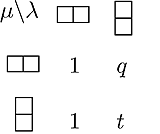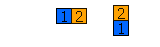HOME: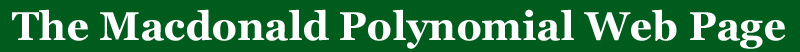BACK: More qt-analogs

# The number of standard tableaux of shape lambda

The number of standard tableaux of a given shape is given by the formula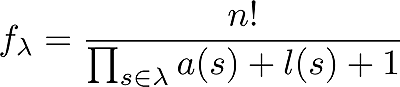where the product runs over all cells s in partition lambda, a(s) represents the arm of the cell s in lambda, l(s) represents the leg of the cell s in lambda
(a(s) + l(s) + 1 is often refered to as the hook of a cell and so this is called the 'hook length formula').

Related to the Macdonald polynomials one has the q,t-coefficients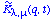and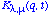referred to as the q,t-Kostka polynomials.

If q and t are both set to 1, we have thatandare both equal to the number of standard tableaux of shape lambda.

### Conjecture:

The polynomials(and) have non-negative integer coefficients and
are a generating function for the number of standard tableaux of shape lambda.
This result (at least the postitivity part) is known forwhen it is of the form (2a1b), (32a1b), (42a1b), (a21b), (a1b)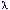when it is of the form (a1b), (a21b), (a31b)
OR the conjugate partition of any of these results.

Note that if q=0 in the polynomial, we have the standard single variable Kostka polynomials which are known to t-count the column strict tableaux of shapeand content.

Below are the tables for the transformed q,t-Kostka polynomialsfor n=2,3 and 4.  You may also find these tables up to n=7 in PDF/poscript and LaTeX form on this web site. and Maple programs by Stembridge and Garsia-Tesler.